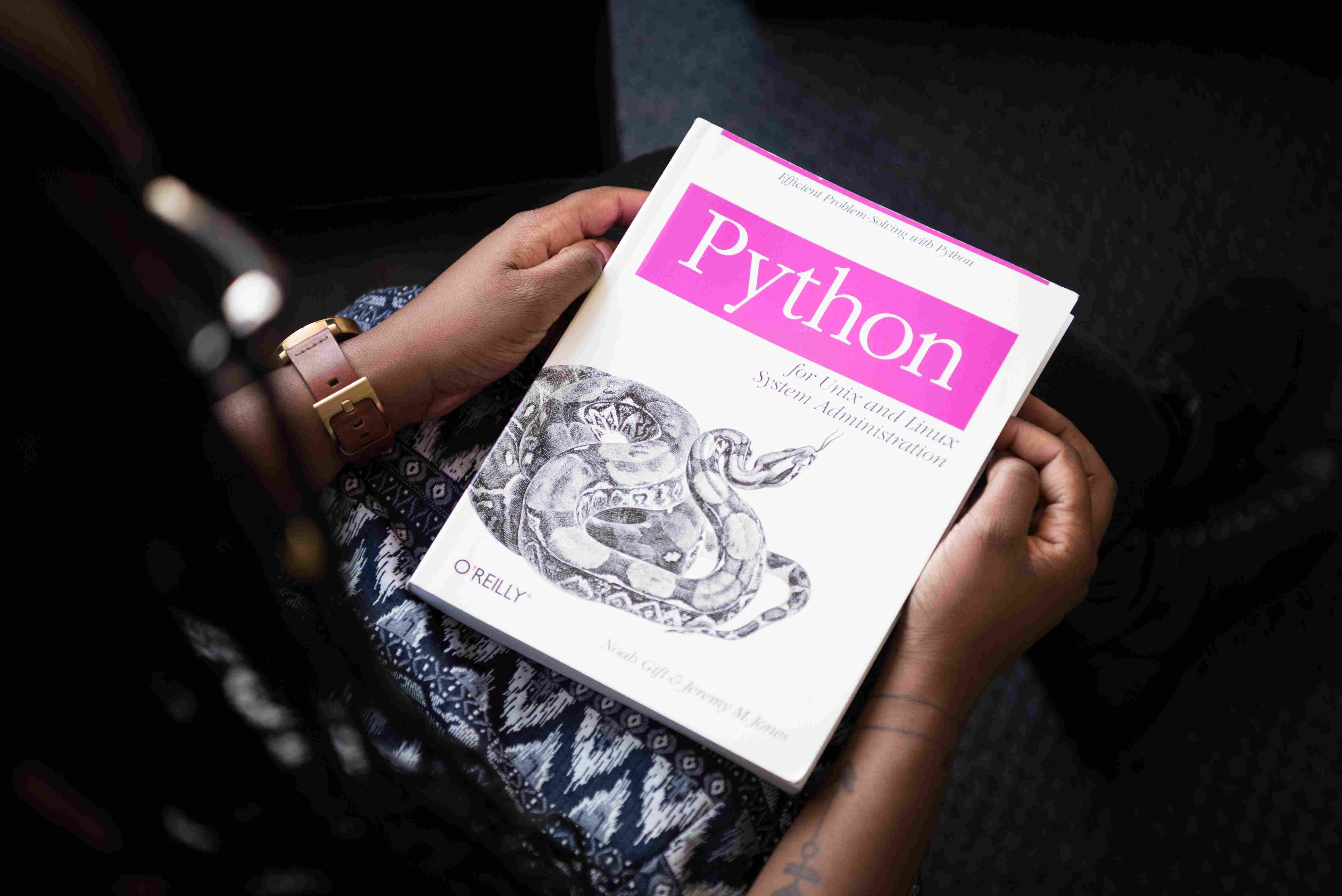# 8 Important Evaluation Metrics in Machine Learning

### Table Of Content

There are different kinds of metrics to evaluate machine learning models. Evaluation metrics are used to measure the quality of the statistical or machine learning model. Let's dive into the topic to learn about the most important metrics to evaluate your machine learning algorithm.

## Confusion Matrix

A confusion matrix is one of the important concepts in the field of machine learning, and so important when it comes to statistical classification problems. A confusion matrix is also known as ERROR MATRIX.

A confusion matrix is usually used to describe the performance of a classification model based on the true values known for a data set.

In simple words, it is a summary of the prediction result made by the classification model.

This is a simple table to denote scores give to the predicted values.

1 0

True PositiveTrue Negative
False NegativeFalse Positive

Learn Web Development - FREE Training

The confusion matrix in itself is not a performance measure but all the performance metrics are dependent on the confusion matrix.

### Terms Associated with Confusion Matrix

1. True Positive (TP): True positive means their actual class value is 1 and the predicted class value is also 1.

Ex: The case where the women are actually pregnant and the model also classifies that she is pregnant.

2. False Positive (FP): False-positive means their actual class value is 0 and the predicted class value is also 1.

Ex: The case where the women are not pregnant and the model classifies that she is pregnant.

3. True Negative (TN): True negative means their actual class value is 0 and the predicted class value is also 0.

Ex: The case where the women are actually not pregnant and the model also classifies that she is not pregnant.

4. False Negative (FN): False-negative means their actual class value is 1 and the predicted class value is also 0.

Ex: The case where the women are actually pregnant and the model also classifies that she is not pregnant.

## Classification Accuracy

Classification accuracy is the most common and simplest metrics that anyone can easily understand. It is defined as the number of correct predictions made to be the developed model.

It can be easily calculated with the help of a confusion matrix.

In simple terms, accuracy means the ratio of several correct predictions to the total number of predictions.

## Precision

Sometimes your classification model may classify based on the most frequent classes. There will also be low accuracy in your classification model since your model doesn’t learn anything and just classify based on the top class.

Therefore, we need a class-specific performance metrics to analyze. Precision is one of them.

Precision can be defined as the division of the total number of correctly classified positive examples by the total number of predicted positive examples.

## Recall

A recall is also known as sensitivity. In simple words recall refers to the percentage of total relevant results correctly classified by the classification model.

Recall is defined as the number of positive samples returned by the custom trained model.

### The relation between recall and precision

High recall, low precision: This means that most of the positive examples are correctly recognized but there are a lot of false positives.

Low recall, high precision: This means that we miss a lot of positive examples but those we predict as positive are indeed positive.

## F1 Score

Based on the classification model we train the priority is given either to recall or precision, but in some classification models, we need both of these metrics to be combined as a single one. F1-Score is a metric that combines both precision and recall.

F1-Score can be defined as the harmonic mean of precision and recall. In simple terms, it's a mathematical average of precision and recall.

F1-Score has an equal and relative contribution of both precision and recall.

## Mean Absolute Error

Absolute error is the total amount of error in any type of measurement made. This is the same for machine learning models too. In many situations, the predicted values may be wrong and the errors must be calculated accurately and more precisely to increase the accuracy of the model.

Absolute error is also called Absolute Accuracy Error.

Mean absolute error is defined as the average of all absolute errors that are calculated based on values predicted by the machine learning model.

## Root Mean Squared Error

RMSE is one of the most popular metrics used for evaluating regression-based machine learning models.

This is an essential evaluation metric since it’s very important to find the average squared error between the predicted values.

RMSE is a quadratic equation scoring rule that measures the average magnitude of the error. It’s the square root of the average of squared differences between prediction and actual observation.

Check out these 7 HTML Tips and Tricks

### Correlation between MAE and RMSE

• Both of these metrics express the average error of the machine learning models.

• These two metrics can range from 0 to ∞ and have the same direction for the errors.

• Both of these metrics are negatively oriented scores, this means that lower score defines better results.

## ROC Curve

ROC Curve stands for “receiver operating characteristic cure”.

ROC Curve is defined as a plot in a graph that shows the performance of a binary classifier as a function of its cut-off threshold.

In simple words, ROC Curve is the once which tells how much a machine learning model is capable of differentiating between the classes.

ROC is a probability curve. It is a representation of the performance of the machine-learning model in a graphical manner.

## Conclusion

So, these are the 8 most important and needed metrics that are so helpful for model evaluation and are to be learned by a data scientist or a machine learning engineer.

Author: B. Vetrichelvan
Email: vetrichelvaninovator@gmail.com